### Home > PC > Chapter 7 > Lesson 7.3.2 > Problem7-113

7-113.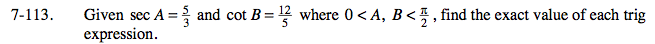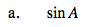Draw a reference triangle in Quadrant 1 for both angles.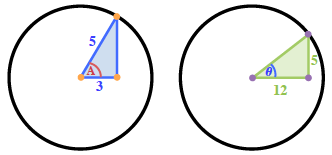Label the 3rd side.
Both are pythagorean triples: 3,4,5 and 5,12,13

Find sin A from the triangle sides.

$\frac{4}{5}$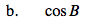Find cos B from the triangle sides.

$\frac{12}{13}$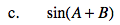Use the sum and difference formula:
sin(A + B) = sinAcosB + cosAsinB

$\text{sin}(A+B)=\frac{4}{5}\cdot \frac{12}{13}+\frac{3}{5}\cdot \frac{5}{13}=$

$\frac{63}{65}$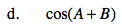Use the sum and difference formula:
cos(A + B) = cosAcosB − sinAsinB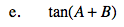$\text{tan}(A+B)=\frac{\text{sin}(A+B)}{\text{cos}(A+B)}$